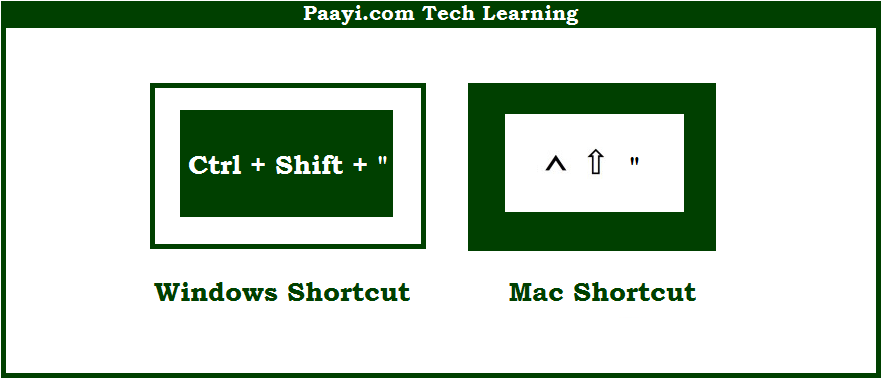# Learn How to Copy Value From Cell Above With Shortcut in Microsoft Excel

Written by | 0 Comments | 644 Views

In this article, you will learn how to Copy Value From Cell Above with a shortcut in Microsoft Excel. You will also get to know the shortcut to Copy Value From Cell Above on Mac. The easy key combinations will make you operate Microsoft Excel smoothly.

Copy Value From Cell Above With Shortcut in Microsoft Excel

Here we will show you how to copy the value from the cell above in Microsoft Excel. Like many other programs Microsoft Excel also uses similar key combinations while using shortcuts.

You can use the key combinations given below to copy the value from the cell above using the shortcut. When you use this shortcut, it will copy the value in the cell above and leaves the cell in edit mode. In case the cell above is the formula, the only value is copied. So, here we go:

## How to Copy Value From Cell Above With Shortcut in Microsoft Excel?It is used to copy values from the cell above in Microsoft Excel. When you use this shortcut, it will copy the value in the cell above and leaves the cell in edit mode. In case the cell above is the formula, the only value is copied. This way you can easily copy the value from the cell above using this shortcut.